# Equivalent fractions

### Equivalent fractions

#### Lessons

In this lesson, we will learn:

• How to find equivalent fractions using models
• How to find equivalent fractions using multiplication (and division)
• How to find fractions in lowest terms (simplest form)

Notes:

• Equivalent fractions are fractions with the same value even though they look different (top and bottoms are not the same numbers)
• This can be proven by showing fraction models
• By using the same whole shape and splitting into different numbers of equal parts, all the shape models have the same proportion of shaded area: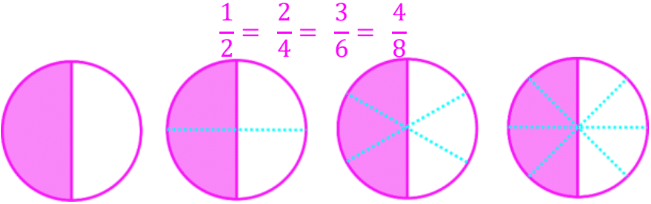• By lining up number lines on top of each other, all the number line models show the dot representing the fractions on the same point along the line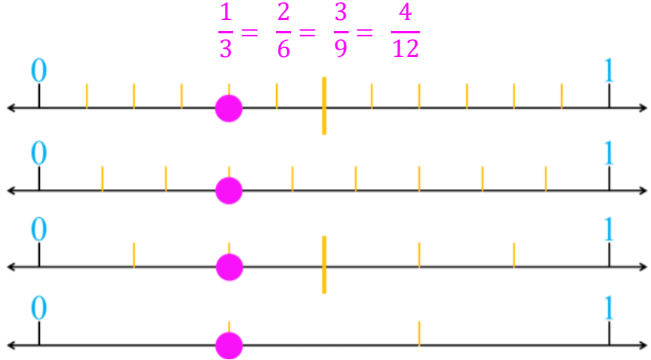• Equivalent fractions have the same value because they take the same fraction (proportion) and multiply BOTH the top and bottom by the same number; the value does not change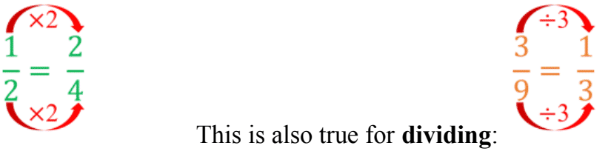• Fractions in lowest terms (or simplest form) are the smallest equivalent fraction
• Divide the top and bottom by the same common factor until you can’t anymore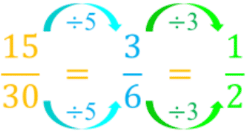• Introduction
Introduction to Equivalent Fractions
a)
What are equivalent fractions?

b)
What are fractions in lowest terms (simplest form)?

• 1.
Equivalent fractions on number lines
Write the equivalent fractions shown on the number line. Use the equal (=) sign in the answer.
a)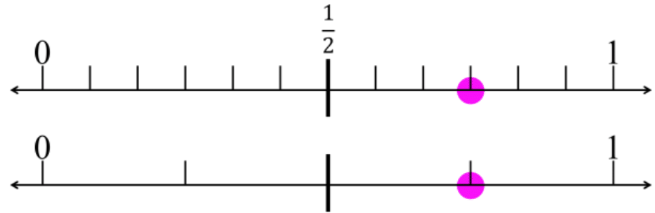b)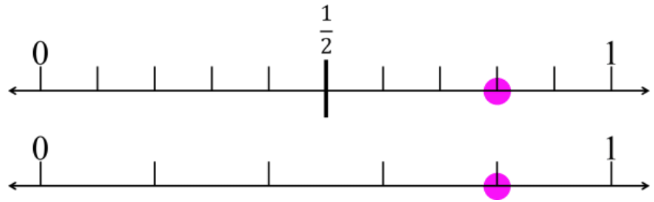• 2.
Equivalent fractions: fill in the blank
Write the missing value to make the fractions equal.
a)
$\large \frac{1}{2}$ = $\large \frac{?}{18}$

b)
$\large \frac{?}{3}$ = $\large \frac{14}{21}$

c)
$\large \frac{1}{4}$ = $\large \frac{25}{?}$

d)
$\large \frac{4}{?}$ = $\large \frac{16}{20}$

• 3.
a)
$\large \frac{2}{20}$ = $\large \frac{?}{?}$

b)
$\large \frac{70}{140}$ = $\large \frac{?}{?}$

c)
$\large \frac{16}{24}$ = $\large \frac{?}{?}$

d)
$\large \frac{27}{36}$ = $\large \frac{?}{?}$